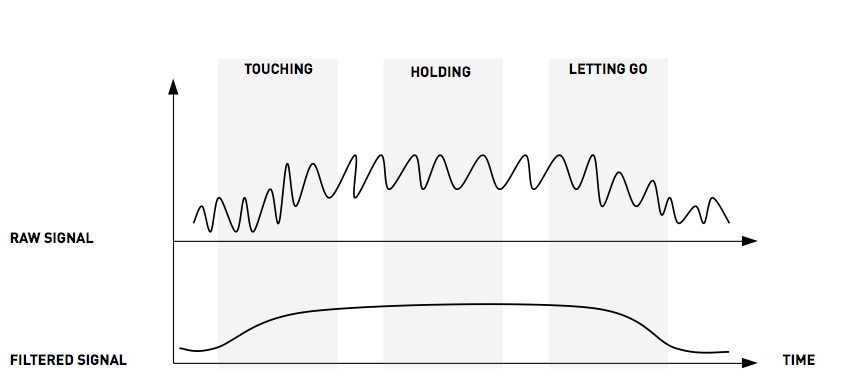Published: October 27, 2014, Edited by: Mads Hobye

# Nonlinear internal complexity with running averageExcerpt from Mads Høbye, Designing for homo Explorens

The input from touch-based systems is most often quite noisy. Within engineering, a whole array of different approaches to this problem exists (Kalman filtering, Bandpass filtering, etc.). When sketching new interaction designs, what is commonly known as running average, has proven to be a good-enough-quick-and-dirty solution to most problems, and moreover, the limitations of the running average can be used as an advantage when working with the aesthetic qualities of interaction design.

When used as a noise filter it takes a noisy input signal, sums it up and gives you an average of it. The trick is that it does not require you to keep all historical data in large tables, run through them and do the average. Instead, it calculates the average on a continuous basis through a relatively simple formula.

The figure above show the noisy signal coming in from the touching/sensing and how it looks (slightly idealised) when it has been filtered by a running average. The formula for the running average looks like the following:

``````float raw = 0;
float filtered = 0;

while (true)
{
filtered = filtered * 0.9 + raw * 0.1;
}
``````

In the above version, the filtered signal is set to 90% of the previous filtered version plus 10% of the raw signal. By tweaking the balance between the two, one can achieve different levels of filtering depending on the amount of noise that needs to be filtered.

As an unwanted side effect, the more filtering the greater delay the filter will generate. The delay presents itself as lowered reaction-time to change in value. This means that the reaction from, e.g., a touch sensor is delayed as a consequence of the filter. Although this would, from an engineering point of view, be considered a flaw in the algorithm, it can generate interesting interaction qualities in the designs. As a consequence of the delay, one can decide the sensitivity of the formula by tweaking the reaction time of the individual patterns. For example, if one wants to create a slow reaction, one can average over a greater amount of data.Caption: Different types of touch calculated through a set of running averages based on a single analog touch sensor.

The figure illustrates different patterns that can be created varying the delay of a running average by adding or subtracting them in real time. The code would look something like this:

``````float raw = 0;
float amountOfTouch = 0;
float amountOfTouchOld = 0;
float amountOfActivity = 0;
float amountOfChange = 0;
float amountOfEnergy = 0;

while (true)
{